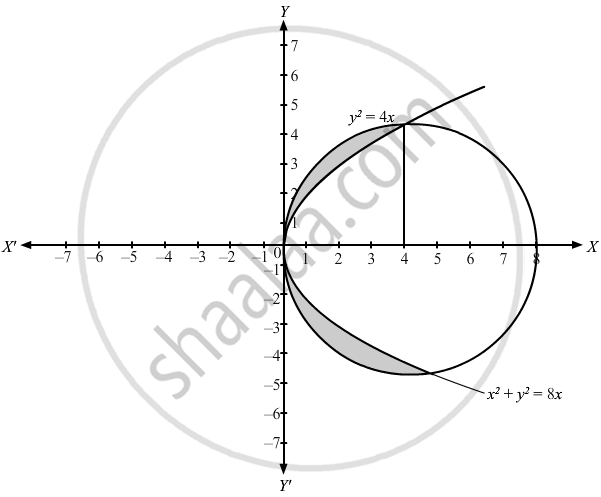Advertisement Remove all ads

# Find the Area of the Region Lying Above X-axis and Included Between the Circle X2 + Y2 = 8x Nd Inside of the Parabola Y 2 = 4 X . - Mathematics

Advertisement Remove all ads
Advertisement Remove all ads
Advertisement Remove all ads
Sum

Find the area of the region lying above x-axis and included between the circle x2 + y2 = 8x nd inside of the parabola y2 = 4x.

Advertisement Remove all ads

#### SolutionThe given equations are x2 + y2 = 8x  ...(1) and y2 = 4x ...(2)
Clearly the equation x2 + y2 = 8x is a circle with centre (4,0) and has a radius 4. Also y2 = 4x is a parabola with vertex at origin and the axis along the x-axis opening in the positive direction.
To find the intersecting points of the curves, we solve both the equation.
∴ x2 + 4x = 8x
⇒ x2 - 4x = 0
⇒ x (x -4) = 0
⇒ x = 0 and x = 4
When x = 0, y = 0
When x = 4,y= ±4

To approximate the area of the shaded region the length = |y_2-y_1| and the width = dx
"A" = int_0^4 |y_2-y_1|dx

= int_0^4 (y_2-y_1)dx ...[∵ y_2>y_1 ∴ |y_2-y_1|= y_2-y_1]

= int_0^4 [sqrt((16-(x-4)^2)) - sqrt(4x)] dx {∴ y_2 = sqrt(16-(x-4)^2) and y_1 = 2sqrt(x)}

= int_0^4 sqrt(16-(x-4)^2) dx - int_0^4 sqrt(4x)  dx

= [((x-4))/2 sqrt(16-(x-4)^2) + 16/2sin^-1((x-4)/(4))]_0^4 -[(4x(3)/(2))/3]_0^4

= [0+0-0-8 sin^-1  ((-4)/(4))] -(4)/(3) xx  4^(3/2)

= (8pi)/2 - 32/3

= 4pi - 32/3

Hence the required area is 4pi - 32/3 square units.

Concept: Area of the Region Bounded by a Curve and a Line
Is there an error in this question or solution?

#### Video TutorialsVIEW ALL 

Advertisement Remove all ads
Share
Notifications

View all notifications

Forgot password?